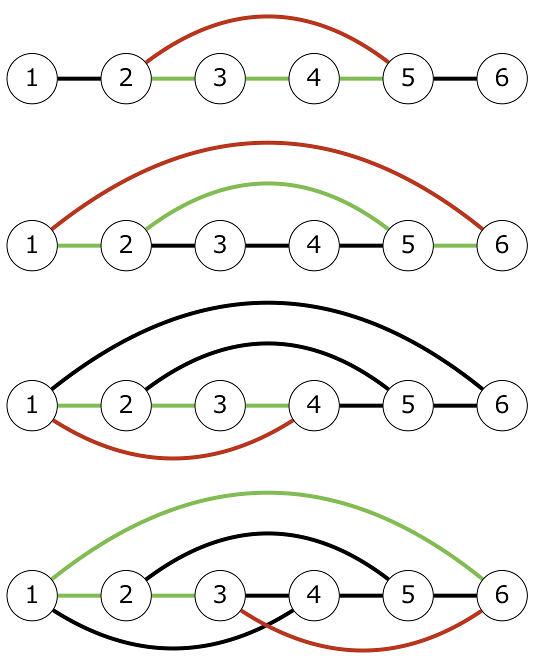# CODE FESTIVAL 2017 qual B

## C - 3 Steps

Time limit時間制限 : 2sec / Memory limitメモリ制限 : 256MB

### 問題文

りんごさんは N 頂点 の連結な無向グラフを持っています。 このグラフにはすでに M 本の辺があり、i 本目の辺は頂点 A_i と頂点 B_i を繋いでいます。

りんごさんは以下の操作を行うことで、辺を追加しようと思っています。

• 操作：頂点 u から辺をちょうど 3 本辿ることによって頂点 v に辿り着けるような u,v (u \neq v) をとり、頂点 u と頂点 v の間に辺を追加する。ただし、すでに頂点 u と頂点 v の間に辺が存在する場合は辺を追加することはできない。

りんごさんが追加できる辺の本数の最大値を求めて下さい。

### 制約

• 2 \leq N \leq 10^5
• 1 \leq M \leq 10^5
• 1 \leq A_i,B_i \leq N
• 多重辺や自己ループは存在しない
• 与えられるグラフは連結である

### 入力

N M
A_1 B_1
A_2 B_2
:
A_M B_M


### 入力例 1

6 5
1 2
2 3
3 4
4 5
5 6


### 出力例 1

4### 入力例 2

5 5
1 2
2 3
3 1
5 4
5 1


### 出力例 2

5


• 頂点 5 と頂点 3 の間に辺を追加する。
• 頂点 5 と頂点 2 の間に辺を追加する。
• 頂点 4 と頂点 1 の間に辺を追加する。
• 頂点 4 と頂点 2 の間に辺を追加する。
• 頂点 4 と頂点 3 の間に辺を追加する。

Score : 500 points

### Problem Statement

Rng has a connected undirected graph with N vertices. Currently, there are M edges in the graph, and the i-th edge connects Vertices A_i and B_i.

Rng will add new edges to the graph by repeating the following operation:

• Operation: Choose u and v (u \neq v) such that Vertex v can be reached by traversing exactly three edges from Vertex u, and add an edge connecting Vertices u and v. It is not allowed to add an edge if there is already an edge connecting Vertices u and v.

Find the maximum possible number of edges that can be added.

### Constraints

• 2 \leq N \leq 10^5
• 1 \leq M \leq 10^5
• 1 \leq A_i,B_i \leq N
• The graph has no self-loops or multiple edges.
• The graph is connected.

### Input

Input is given from Standard Input in the following format:

N M
A_1 B_1
A_2 B_2
:
A_M B_M


### Output

Find the maximum possible number of edges that can be added.

### Sample Input 1

6 5
1 2
2 3
3 4
4 5
5 6


### Sample Output 1

4


If we add edges as shown below, four edges can be added, and no more.### Sample Input 2

5 5
1 2
2 3
3 1
5 4
5 1


### Sample Output 2

5


Five edges can be added, for example, as follows:

• Add an edge connecting Vertex 5 and Vertex 3.
• Add an edge connecting Vertex 5 and Vertex 2.
• Add an edge connecting Vertex 4 and Vertex 1.
• Add an edge connecting Vertex 4 and Vertex 2.
• Add an edge connecting Vertex 4 and Vertex 3.